Lec 22 - Dimensions

Wednesday, February 29, 2012

9:30 AM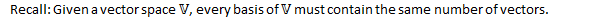We can interpret a basis as:

1. A minimal spanning set of the vector space
1. A maximal linearly independent set of the vector space.

Definition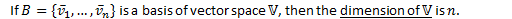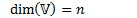Eg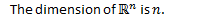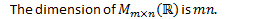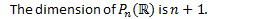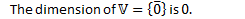Eg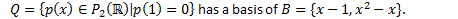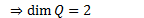Theorem: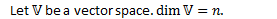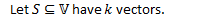1.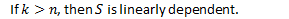1.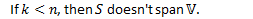1.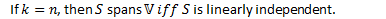Proof of 3: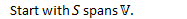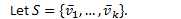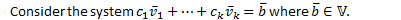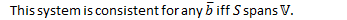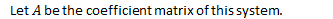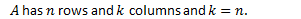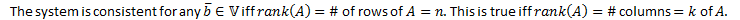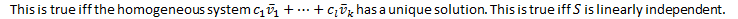Q.E.D.Theorem: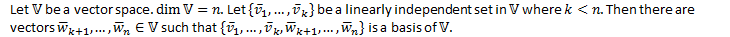Proof: A7

Eg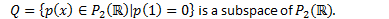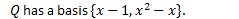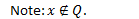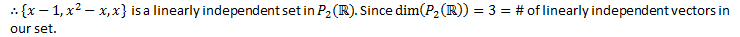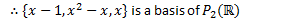Eg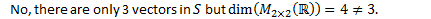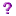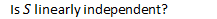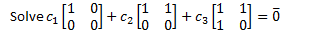This gives a system of linear equations: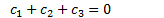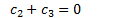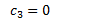Row reduce the coefficient matrix: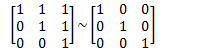Since rank of coefficient matrix equals the # of columns and the system Is consistent, the system has a unique solution.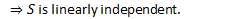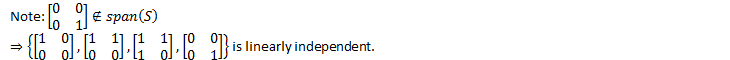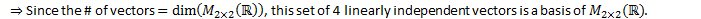Coordinates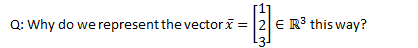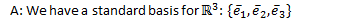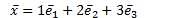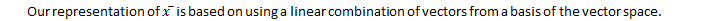But we could also use another basis.

Eg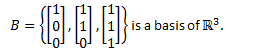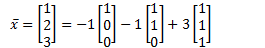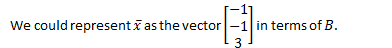Definition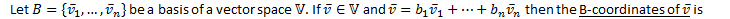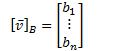Note: Order of basis vectors matters.

Eg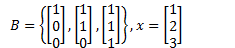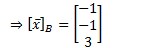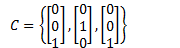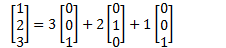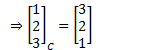Eg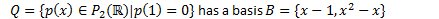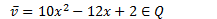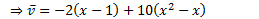Created by Tim Pei with Microsoft OneNote 2010
One place for all your notes and information